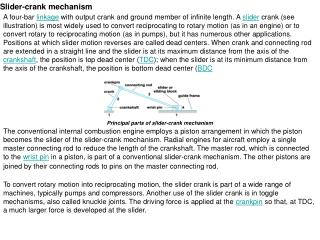DownloadDownload PresentationSlider-crank mechanism

# Slider-crank mechanism

Télécharger la présentation## Slider-crank mechanism

- - - - - - - - - - - - - - - - - - - - - - - - - - - E N D - - - - - - - - - - - - - - - - - - - - - - - - - - -
##### Presentation Transcript

1. Slider-crank mechanism A four-bar linkage with output crank and ground member of infinite length. A slider crank (see illustration) is most widely used to convert reciprocating to rotary motion (as in an engine) or to convert rotary to reciprocating motion (as in pumps), but it has numerous other applications. Positions at which slider motion reverses are called dead centers. When crank and connecting rod are extended in a straight line and the slider is at its maximum distance from the axis of the crankshaft, the position is top dead center (TDC); when the slider is at its minimum distance from the axis of the crankshaft, the position is bottom dead center (BDC Principal parts of slider-crank mechanism The conventional internal combustion engine employs a piston arrangement in which the piston becomes the slider of the slider-crank mechanism. Radial engines for aircraft employ a single master connecting rod to reduce the length of the crankshaft. The master rod, which is connected to the wrist pin in a piston, is part of a conventional slider-crank mechanism. The other pistons are joined by their connecting rods to pins on the master connecting rod. To convert rotary motion into reciprocating motion, the slider crank is part of a wide range of machines, typically pumps and compressors. Another use of the slider crank is in toggle mechanisms, also called knuckle joints. The driving force is applied at the crankpin so that, at TDC, a much larger force is developed at the slider.

3. Crankshaft geometry: Definitions l = rod length (distance between piston pin and crank pin)r = crankradius (distance between crank pin and crank center, i.e. half stroke)A = crank angle (from cylinderbore centerline at TDC)x= piston pin position (upward from crank center along cylinder bore centerline)v = piston pin velocity (upward from crank center along cylinder bore centerline)a = piston pin acceleration (upward from crank center along cylinder bore centerline)ω = crank angular velocity in rad/s Diagram showing geometric layout of piston pin, crank pin and crank center

4. Angular velocity: The crankshaftangular velocity is related to the engine revolutions per minute (RPM): Triangle relation: As shown in the diagram, the crank pin, crank center and piston pin form triangle NOP.By the cosine law it is seen that: Equations with respect to angular position (Angle Domain): The equations that follow describe the reciprocating motion of the piston with respect to crank angle.Example graphs of these equations are shown below. Position: Position with respect to crank angle (by rearranging the triangle relation):

5. Velocity: Velocity with respect to crank angle (take first derivative, using the chain rule): Acceleration: Acceleration with respect to crank angle (take second derivative, using the chain rule and the quotient rule):

6. Example graph of piston motion: The graph shows x, x', x" wrt to crank angle for various half strokes, where L = rod length (l) and R = half stroke (r): The vertical axis units are inches for position, [inches/rad] for velocity, [inches/rad²] for acceleration.The horizontal axis units are crank angle degrees.

7. Experiment # 1: Slider crank mechanism Learning Objectives / Experiments: - Crank drive with fixed cylinder - Crank drive with swivelling cylinder Exp. Procedure and Readings to be taken: 1- select and adjust certain r and L. 2- calculate the ratio: λ = r / L 3- measure the piston displacement (x) and plot graph (x) against the crank angle (θc).

8. 4- The piston traveling stroke: range: Xmax – Xmin =______________________________________ 5- graph the function 6- graph differentiating piston displacement (V) versus crank angle (θ)